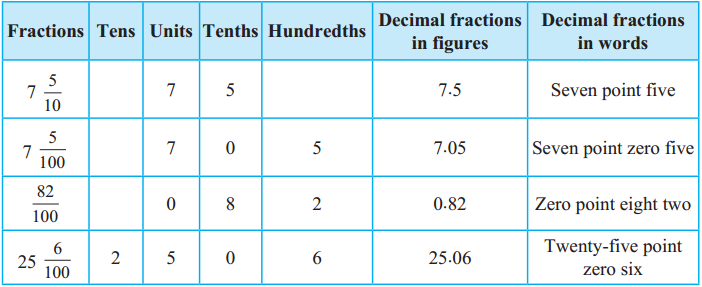# Maharashtra Board Class 5 Maths Solutions Chapter 9 Decimal Fractions Problem Set 36

Balbharti Maharashtra Board Class 5 Maths Solutions Chapter 9 Decimal Fractions Problem Set 36 Textbook Exercise Important Questions and Answers.

## Maharashtra State Board Class 5 Maths Solutions Chapter 9 Decimal Fractions Problem Set 36

Write the following mixed fractions in decimal form and read them aloud.

$$\text { (1) } 3 \frac{9}{10}$$
3.9, Three-point nine.$$\text { (2) } 1 \frac{4}{10}$$
1.4, One point four.

$$\text { (3) } 5 \frac{3}{10}$$
5.3, Five-point three.

$$\text { (4) } \frac{8}{10}$$
0.8, Zero points eight.

$$\text { (5) } \frac{7}{10}$$
0.5, Zero points five.Hundredths

If $$\frac{1}{10}$$ is divided into 10 equal parts, each part becomes $$\frac{1}{100}$$ or one hundredth. Therefore, note that 1 tenth =10 hundredths, or 0.1=0.10. By multiplying $$\frac{1}{100}$$ by 10 we get $$\frac{10}{100}$$ = $$\frac{1}{10}$$. Therefore, it is possible to create a hundredths place next to the tenths place. After creating a hundredths place we can write $$\frac{14}{100}$$ as 0.14.

$$\frac{14}{100}=\frac{10+4}{100}=\frac{10}{100}+\frac{4}{100}=\frac{1}{10}+\frac{4}{100}$$ meaning that when writing $$\frac{14}{100}$$ in decimal form, 1 is written in the tenths place and 4 is written in the hundredths place. This fraction is written as 0.14 and is read as ‘zero point one four’. Similarly, 6 $$\frac{57}{100}$$ is written as 6.57 and 50 $$\frac{71}{100}$$ is written as 50.71.

While writing $$\frac{3}{100}$$ in decimal form, we must remember that there is no number in the tenths place and so, we put 0 in that place, which means that $$\frac{3}{100}$$ is written as 0.03.

Study how the decimal fractions in the table below are written and read.$$\text { (1) } 4 \frac{6}{10}$$
4.6, Four point six. 7

$$\text { (2) } 4 \frac{6}{10}$$
2.7, Two point seven.

$$\text { (3) } 4 \frac{6}{10}$$
$$\text { (4) } 4 \frac{6}{10}$$$$\text { (5) } 4 \frac{6}{10}$$